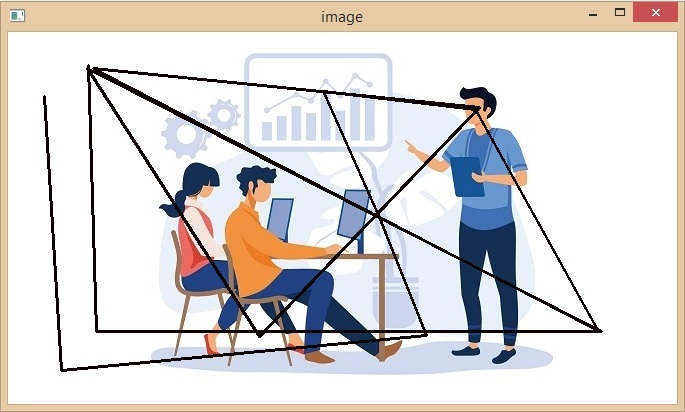# Connect new point to the previous point on a image with a straight line in OpenCV-Python

We might have to draw lines on an image for various purposes like drawing, scribbling, tracking the movements of a point etc. so it is necessary to know how to connect 2 points in image processing.

OpenCV is a library of programming functions primarily for real-time computer vision. The library is cross-platform and licensed as free and open source software under the Apache License.

In this article, we will learn how we can connect a new point to the previous point on an image with a straight line using OpenCV-Python.

## Connecting a Points on with the Previous One

Our task is to connect the current coordinate position on the image on which the mouse click is performed with the previous point.

Before starting the steps to connect the points we should know how we will be doing it. An image is made up of many pixels which can be specified by coordinates we can select any 2 points on this image and they both will have some coordinates by connecting these 2 points we can easily draw a line. But we need to have those coordinates with us.

To draw a straight line between two points on an image we can use the OpenCV cv2.line(). The syntax of this method is –

cv2.line(img, pt1, pt2, color, thickness)


To draw lines between each mouse click you need to follow the steps given below –

### Step 1: Importing the Required Libraries

The first step is to import the required libraries. To open and read and manipulate the images we will be using the Open CV library

import cv2
import numpy as np


### Step 2: Reading the Image

We can use the cv2.imread() function to read an image from a file. In the following snippet, we have read an image from a file and displayed it using the cv2.imshow function.

# Read the image

# Display the image
cv2.imshow('Image', img)
cv2.waitKey(0)


### Step 3: Declaring a List Containing all the Points

To keep a track of all the points visited we will create a list in which we will append all the points on the images where we clicked our mouse

Point = []


### Step 4: Defining the Mouse Click Event

The next step is to define the action we need to perform whenever the mouse is clicked anywhere on the screen.

• We will first add the coordinates of the point we clicked in the points list.

• Then we will create a small dot to show the point where we clicked the image.

• If we click the image for the first time, there is no need to draw a line.

• But after that we need to draw a line between the last 2 points in the points list. Then we will show the new image.

• We will add this function as callback to mouse events.

In the following snippet, the cv2.setMouseCallback function captures the mouse events and then appends the position of the mouse click when the left mouse button is pressed and stores the coordinates of the new point in the points list. Then the last 2 points available in the points list are connected using a straight line.

Here we have used the cv2.line() function to draw a line between the previous point and the new point. The function takes as input the coordinates of the two points and the color and thickness of the line. We have then displayed the image with the line using the cv2.imshow function.

# Define a callback function to capture mouse events
def mouse_callback(event, x, y, flags, param):
if event == cv2.EVENT_LBUTTONDOWN:
points.append((x,y))
cv2.circle(img,(x,y), 1, (100, 100, 100), -1)
if len(points) >= 2:
cv2.line(img, points[-1], points[-2], (0, 0, 10), 2)
cv2.imshow('image', img)

# Set the callback function for mouse events
cv2.setMouseCallback('Image', mouse_callback)

# Wait for the user to click on the image
cv2.waitKey(0)


### Step 6: Closing all the Windows

Finally, we need to destroy all the windows using the destroyAllWindows() function –

Cv2.destroyAllWindows()


## Example

Here is the complete code to connect a new point to the previous point on an image with a straight line using OpenCV-Python −

import cv2
import numpy as np
cv2.imshow('image',img)
points = []
def click_event(event, x, y, flags, params):
if event == cv2.EVENT_LBUTTONDOWN:
points.append((x,y))
cv2.circle(img,(x,y), 1, (100, 100, 100), -1)
if len(points) >= 2:
cv2.line(img, points[-1], points[-2], (0, 0, 10), 2)
cv2.imshow('image', img)
cv2.setMouseCallback('image', click_event)
cv2.waitKey(0)
cv2.destroyAllWindows()


## Output## Conclusion

In this article we saw how we can connect a new point to a previous point on an image with a straight line using OpenCV library of Python language. We went through all the steps needed to read the image, defining the previous and new point and then connecting the points with a straight line. We came to know about the Opencv library of python and its applications in image processing.

Updated on: 20-Apr-2023

338 Views